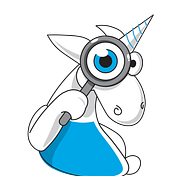# Top 10 C# projects errors found in 2016

`void IBackgroundTask.Sweep(){   ....  // Don't flood the database with progress updates;   // Limit it to every 5 seconds.  if ((_clock.UtcNow - lastUpdateUtc).Seconds >= 5)  {     ....  }}`
`string rev1 = "";string rev2 = "";var revisions = RevisionGrid.GetSelectedRevisions();if (revisions.Count > 0){  rev1 = ....;  rev2 = ....;  ....}elseif (string.IsNullOrEmpty(rev1) || string.IsNullOrEmpty(rev2)) // <={    MessageBox.Show(....);    return;}`
`public void SetPrices(....){    UInt32 a0 = _choice.GetPrice0();    UInt32 a1 = _choice.GetPrice1();    UInt32 b0 = a1 + _choice2.GetPrice0();  // <=    UInt32 b1 = a1 + _choice2.GetPrice1();    ....}`
`private object Aggregate(....){  ....  if (name != "Min" || name != "Max")  {    throw new ArgumentException(      "Can only use aggregate min or max methods on properties       which are datetime");  }  ....}`
`public static void Convert(float[][] from, short[][] to){  for (int i = 0; i < from.Length; i++)    for (int j = 0; i < from.Length; j++)      to[i][j] = (short)(from[i][j] * (32767f));}`
`public JaggedSingularValueDecompositionF(....){  ....  if ((k < nct) & (s[k] != 0.0))  ....}`
`void WriteSecurityDeclarationArgument(CustomAttributeNamedArgument na){  ....  output.Write("string('{0}')",    NRefactory.CSharp.TextWriterTokenWriter.ConvertString((string)na.Argument.Value).Replace("'", "\'"));  ....}`
`static class Profiler{  [ThreadStatic]  private static Stopwatch timer = new Stopwatch();  ....}`
`button_pressed_highlight = use_system_colors ?                           Color.FromArgb (150, 179, 225) :                            Color.FromArgb (150, 179, 225);`
`public void ProcessFiles(....){  ....  int RowsCount =     DynamicErrorListControl.Instance.Plog.NumberOfRows;  if (RowsCount > 20000)    DatatableUpdateInterval = 30000; //30s  else if (RowsCount > 100000)    DatatableUpdateInterval = 60000; //1min  else if (RowsCount > 200000)    DatatableUpdateInterval = 120000; //2min  ....}`

--

--## Unicorn Developer

The developer, the debugger, the unicorn. I know all about static analysis and how to find bugs and errors in C, C++, C#, and Java source code.